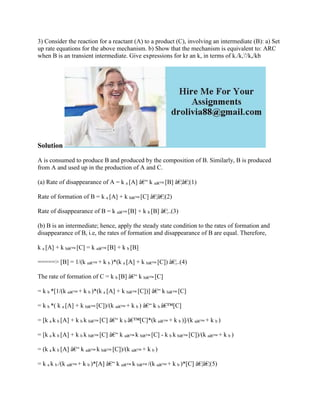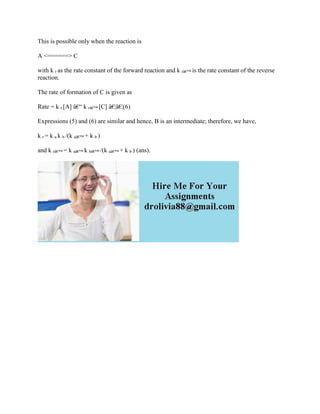Diese Präsentation wurde erfolgreich gemeldet.

# 3) Consider the reaction for a reactant (A) to a product (C)- involvin.docx

Anzeige
Anzeige
Anzeige
Anzeige
Anzeige
Anzeige
Anzeige
Anzeige
Anzeige
Anzeige
Anzeige×

1 von 2 Anzeige

# 3) Consider the reaction for a reactant (A) to a product (C)- involvin.docx

3) Consider the reaction for a reactant (A) to a product (C), involving an intermediate (B): a) Set up rate equations for the above mechanism. b) Show that the mechanism is equivalent to: ARC when B is an transient intermediate. Give expressions for kr an k, in terms of k./k,\'/k,/kb
Solution
A is consumed to produce B and produced by the composition of B. Similarly, B is produced from A and used up in the production of A and C.
(a) Rate of disappearance of A = k a [A] â€“ k aâ€™ [B] â€¦â€¦(1)
Rate of formation of B = k a [A] + k bâ€™ [C] â€¦â€¦(2)
Rate of disappearance of B = k aâ€™ [B] + k b [B] â€¦..(3)
(b) B is an intermediate; hence, apply the steady state condition to the rates of formation and disappearance of B, i.e, the rates of formation and disappearance of B are equal. Therefore,
k a [A] + k bâ€™ [C] = k aâ€™ [B] + k b [B]
=====> [B] = 1/(k aâ€™ + k b )*(k a [A] + k bâ€™ [C]) â€¦..(4)
The rate of formation of C = k b [B] â€“ k bâ€™ [C]
= k b *[1/(k aâ€™ + k b )*(k a [A] + k bâ€™ [C])] â€“ k bâ€™ [C]
= k b *( k a [A] + k bâ€™ [C])/(k aâ€™ + k b ) â€“ k b â€™[C]
= [k a k b [A] + k b k bâ€™ [C] â€“ k b â€™[C]*(k aâ€™ + k b )]/(k aâ€™ + k b )
= [k a k b [A] + k b k bâ€™ [C] â€“ k aâ€™ k bâ€™ [C] - k b k bâ€™ [C])/(k aâ€™ + k b )
= (k a k b [A] â€“ k aâ€™ k bâ€™ [C])/(k aâ€™ + k b )
= k a k b /(k aâ€™ + k b )*[A] â€“ k aâ€™ k bâ€™ /(k aâ€™ + k b )*[C] â€¦â€¦(5)
This is possible only when the reaction is
A <======> C
with k r as the rate constant of the forward reaction and k râ€™ is the rate constant of the reverse reaction.
The rate of formation of C is given as
Rate = k r [A] â€“ k râ€™ [C] â€¦â€¦(6)
Expressions (5) and (6) are similar and hence, B is an intermediate; therefore, we have,
k r = k a k b /(k aâ€™ + k b )
and k râ€™ = k aâ€™ k bâ€™ /(k aâ€™ + k b ) (ans).
.

3) Consider the reaction for a reactant (A) to a product (C), involving an intermediate (B): a) Set up rate equations for the above mechanism. b) Show that the mechanism is equivalent to: ARC when B is an transient intermediate. Give expressions for kr an k, in terms of k./k,\'/k,/kb
Solution
A is consumed to produce B and produced by the composition of B. Similarly, B is produced from A and used up in the production of A and C.
(a) Rate of disappearance of A = k a [A] â€“ k aâ€™ [B] â€¦â€¦(1)
Rate of formation of B = k a [A] + k bâ€™ [C] â€¦â€¦(2)
Rate of disappearance of B = k aâ€™ [B] + k b [B] â€¦..(3)
(b) B is an intermediate; hence, apply the steady state condition to the rates of formation and disappearance of B, i.e, the rates of formation and disappearance of B are equal. Therefore,
k a [A] + k bâ€™ [C] = k aâ€™ [B] + k b [B]
=====> [B] = 1/(k aâ€™ + k b )*(k a [A] + k bâ€™ [C]) â€¦..(4)
The rate of formation of C = k b [B] â€“ k bâ€™ [C]
= k b *[1/(k aâ€™ + k b )*(k a [A] + k bâ€™ [C])] â€“ k bâ€™ [C]
= k b *( k a [A] + k bâ€™ [C])/(k aâ€™ + k b ) â€“ k b â€™[C]
= [k a k b [A] + k b k bâ€™ [C] â€“ k b â€™[C]*(k aâ€™ + k b )]/(k aâ€™ + k b )
= [k a k b [A] + k b k bâ€™ [C] â€“ k aâ€™ k bâ€™ [C] - k b k bâ€™ [C])/(k aâ€™ + k b )
= (k a k b [A] â€“ k aâ€™ k bâ€™ [C])/(k aâ€™ + k b )
= k a k b /(k aâ€™ + k b )*[A] â€“ k aâ€™ k bâ€™ /(k aâ€™ + k b )*[C] â€¦â€¦(5)
This is possible only when the reaction is
A <======> C
with k r as the rate constant of the forward reaction and k râ€™ is the rate constant of the reverse reaction.
The rate of formation of C is given as
Rate = k r [A] â€“ k râ€™ [C] â€¦â€¦(6)
Expressions (5) and (6) are similar and hence, B is an intermediate; therefore, we have,
k r = k a k b /(k aâ€™ + k b )
and k râ€™ = k aâ€™ k bâ€™ /(k aâ€™ + k b ) (ans).
.

Anzeige
Anzeige

### 3) Consider the reaction for a reactant (A) to a product (C)- involvin.docx

1. 1. 3) Consider the reaction for a reactant (A) to a product (C), involving an intermediate (B): a) Set up rate equations for the above mechanism. b) Show that the mechanism is equivalent to: ARC when B is an transient intermediate. Give expressions for kr an k, in terms of k./k,'/k,/kb Solution A is consumed to produce B and produced by the composition of B. Similarly, B is produced from A and used up in the production of A and C. (a) Rate of disappearance of A = k a [A] â€“ k aâ€™ [B] â€¦â€¦(1) Rate of formation of B = k a [A] + k bâ€™ [C] â€¦â€¦(2) Rate of disappearance of B = k aâ€™ [B] + k b [B] â€¦..(3) (b) B is an intermediate; hence, apply the steady state condition to the rates of formation and disappearance of B, i.e, the rates of formation and disappearance of B are equal. Therefore, k a [A] + k bâ€™ [C] = k aâ€™ [B] + k b [B] =====> [B] = 1/(k aâ€™ + k b )*(k a [A] + k bâ€™ [C]) â€¦..(4) The rate of formation of C = k b [B] â€“ k bâ€™ [C] = k b *[1/(k aâ€™ + k b )*(k a [A] + k bâ€™ [C])] â€“ k bâ€™ [C] = k b *( k a [A] + k bâ€™ [C])/(k aâ€™ + k b ) â€“ k b â€™[C] = [k a k b [A] + k b k bâ€™ [C] â€“ k b â€™[C]*(k aâ€™ + k b )]/(k aâ€™ + k b ) = [k a k b [A] + k b k bâ€™ [C] â€“ k aâ€™ k bâ€™ [C] - k b k bâ€™ [C])/(k aâ€™ + k b ) = (k a k b [A] â€“ k aâ€™ k bâ€™ [C])/(k aâ€™ + k b ) = k a k b /(k aâ€™ + k b )*[A] â€“ k aâ€™ k bâ€™ /(k aâ€™ + k b )*[C] â€¦â€¦(5)
2. 2. This is possible only when the reaction is A <======> C with k r as the rate constant of the forward reaction and k râ€™ is the rate constant of the reverse reaction. The rate of formation of C is given as Rate = k r [A] â€“ k râ€™ [C] â€¦â€¦(6) Expressions (5) and (6) are similar and hence, B is an intermediate; therefore, we have, k r = k a k b /(k aâ€™ + k b ) and k râ€™ = k aâ€™ k bâ€™ /(k aâ€™ + k b ) (ans).